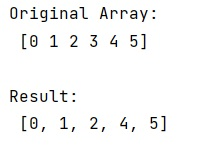# How to index every element in a list except one?

Learn, how to index every element in a list except one in Python?
Submitted by Pranit Sharma, on December 24, 2022

NumPy is an abbreviated form of Numerical Python. It is used for different types of scientific operations in python. Numpy is a vast library in python which is used for almost every kind of scientific or mathematical operation. It is itself an array which is a collection of various methods and functions for processing the arrays.

## Indexing every element in a list except one

Suppose we are given a NumPy array, and if we use the indexing on this, we will get the specific elements according to the positions of the elements.

We need to find a way so we will return the whole list with indexing except for a specific value.

We will use the list comprehension to make a copy of the original array without the specific element such that we will return all the elements while indexing except that specific element.

This is a very general technique and can be used with all the tables including lists also.

Let us understand with the help of an example,

## Python code to index every element in a list except one

```# Import numpy
import numpy as np

# Creating a numpy array
arr = np.array([0, 1, 2, 3, 4, 5])

# Display original array
print("Original Array:\n",arr,"\n")

# Defining an empty list
res = []

# Looping over arr to make a copy
# without the specific element
for i in range(len(arr)):
if i!=3:
res.append(arr[i])
else:
continue

# Display result
print("Result:\n",res,"\n")
```

Output: